Technical Information Site of Power Supply Design

2017.05.25 Transfer Function

# The Reason Why Compensation Ramp Slope Must Be At Least 1/2 Down Slope

DC/DC Converters: Sharing of Transfer Functions among Control Systems

In the previous section, we determined an analytic solution for subharmonic oscillation. In an actual current (peak-current) mode DC/DC converter, a method known as "slope compensation" is used to address subharmonic oscillation. Slope compensation is probably best known as a method in which a right-downward ramp wave voltage is applied to the current threshold generated by an error amplifier to accomplish stabilization. It is probably also well known that "the slope of the compensation ramp must be greater than 1/2 the down-slope of the current waveform".

Here, we will derive an equation that indicates why the slope of the slope compensation must be at least 1/2 of the current waveform down-slope.

As explained in the previous section, the coil (inductor) current waveform of a current-mode step-down converter is as shown in Fig. 10.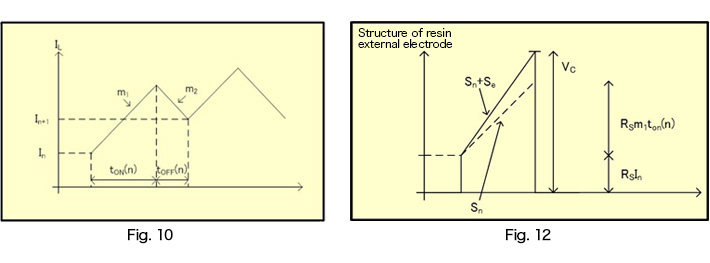Fig. 12 shows a PWM input waveform for a case in which compensation is considered for the waveform of Fig. 10.
Let the current sense gain be RS, the slope due to the ripple current be Sn, the slope for slope compensation be Se, and the down slope be Sp. After ton, the PWM input peak voltage (Vc) can be expressed as in equation 3-23.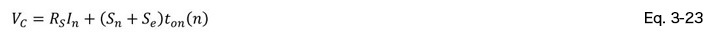Similarly to when equation 3-20 was derived in the previous section, calculations are performed to obtain equation 3-24.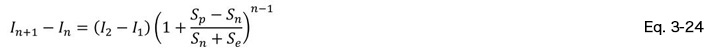Similarly to the previous section, {In+1 - In} is a geometric progression, and so the condition for not causing subharmonic oscillation is that the geometric progression converges to 0 as n → ∞. That is, the condition expressed by equation 3-25 below must obtain: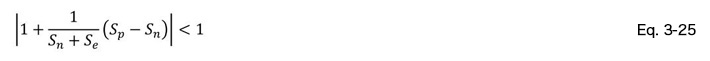From equation 3-25, we obtain equation 3-26.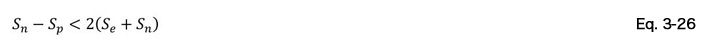In equation 3-26, the strictest condition for Se is when Sn = 0. Equation 3-27 gives Se, that is, the slope in slope compensation, for this condition.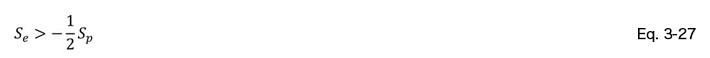As indicated in equation 3-27, the slope Se in slope compensation is at least 1/2 the down slope Sp.

With this explanation of compensation ramps, we conclude our discussion of "slope transfer functions", in succession to "Voltage Mode Transfer Functions", "Current Mode Transfer Functions", "Derivation of Fm", and "Analytic Solution for Subharmonic Oscillation".